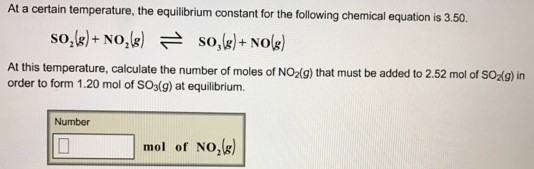# Problem: At a certain temperature, the equilibrium constant for the following chemical equation is 3.50. SO2(g) + NO2(g) ⇌ SO3(g) + NO(g) At this temperature, calculate the number of moles of NO2(g) that must be added to 2.52 mol of SO2(g) in order to form 1.20 mol of SO3(g) at equilibrium.

###### FREE Expert Solution
96% (349 ratings)###### Problem Details

At a certain temperature, the equilibrium constant for the following chemical equation is 3.50.

SO2(g) + NO2(g) ⇌ SO3(g) + NO(g)

At this temperature, calculate the number of moles of NO2(g) that must be added to 2.52 mol of SO2(g) in order to form 1.20 mol of SO3(g) at equilibrium.